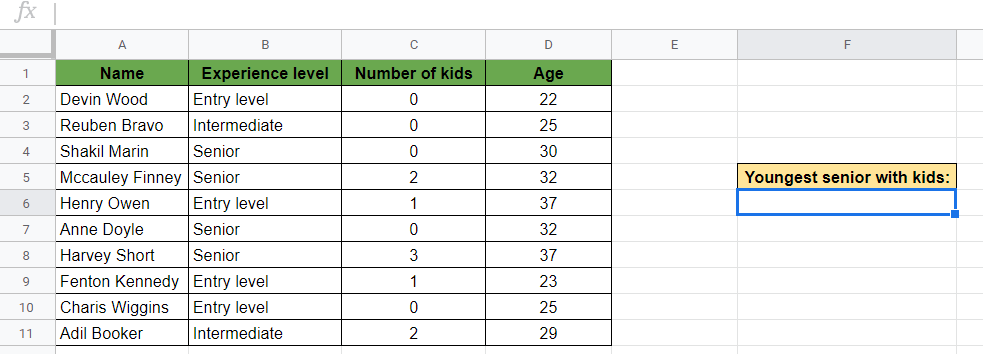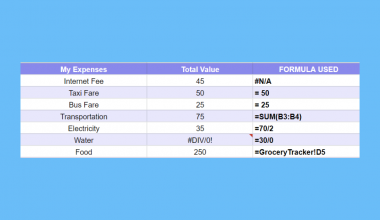# How to Use MINIFS Function in Google Sheets

The MINIFS function in Google Sheets is useful to return the minimum value in a range of cells, filtered by a set of criteria.

The purpose of the `MINIFS` function is to get the minimum value based on one or more conditions. It does this by combining the `MIN` function to find the smallest value and the `IFS` function to filter by a set of expressions.

Let’s take an example.

Say we want to find the youngest person in a company based on some conditions — for example, the youngest employee with senior-level experience.

After that, we might add even more conditions to filter by. We would like to find the youngest senior-level employee that has at least one child.

So how can we do that?

Simple. The `MINIFS` function can find the smallest value in a data set after applying one or more criteria.

Before we dive into a few examples, let’s get to know how the MINIFS function in Google Sheets works.

## The Anatomy of the MINIFS Function

The syntax of a function specifies how we should use the function. For the `MINIFS` function, the syntax looks like this:

`=MINIFS(range, criteria_range1, criterion1, [criteria_range2, criterion2, …])`

Let’s break this syntax down and understand what these terms mean:

• `=` the equal sign is how we start each and every function in Google Sheets.
• `MINIFS` is our function. We will have to add the subsequent variables into it for it to work.
• `range` is the range of cells from which we want to find the minimum value. It is a required field and should contain numeric values.
• `criteria_range1` is the range of cells over which we want to evaluate a condition. It can be the same range as the original `range` or any other range of the same size.
• `criterion1` is the condition we want to apply to the selected `criteria_range1`. The criterion will filter which cells should be considered to find the minimum.
• `criteria_range2`, `criterion2` , `...` are optional additional ranges and their associated criteria.

### ⚠️ A Few Notes to Make Your MINIFS Function Work Perfectly

1. The shape and size of the range and all the criteria ranges must be equal. Otherwise, you will get an error value (#VALUE! error).
2. The `MINIFS` function will return 0 if none of the criteria is satisfied.
3. While the range of the `MINIFS` function must contain numeric values to get a minimum value, the criteria can be applied to dates, numbers, and text values as well.
4. It supports the use of logical operators (>,<,<>,=) and wildcards (*,?) in the criteria. The wildcards can be used to define partial matching.

Now let’s jump right into some examples where we can write our own `MINIFS` function.

## A Real Example of Using MINIFS Function

Take a look at the examples below to see how to use the MINIFS function in Google Sheets.

### MINIFS Function with One Condition

Let’s start with a `MINIFS` function with one condition.

We have the list of employees and their data including their age, experience level and the number of kids. We would like to find out what is the minimum age among the senior-level employees.

The picture below shows how we looked for this minimum age using the `MINIFS` function.We used one condition to filter by. When finding the minimum of the ages, we only wanted to consider the rows where the “Experience level” is “Senior”.

The formula is as follows:

`=MINIFS(D2:D11,B2:B11,"Senior")`

Here is what this example does:

• Firstly, we selected a cell where we wanted to write our `MINIFS` formula. Here we wrote the formula in cell F3.
• We wrote a `MINIFS` function with the three required variables. The first variable is the range, that is the range of cells from which we want to find the minimum value. Here it’s the range D2:D11 where the ages are.
• Then, we added the range of our criterion. As we are filtering for experience levels, the range of the condition is B2:B11.
• After that, we added the condition itself. We want the experience level to be “Senior”, so this is our third argument in the function.
• As a result, we got 30 which is the youngest age among the employees that matched our condition.

Easy, right? 😀

Feel free to make a copy of the spreadsheet using the link I have attached below and have a try:

### MINIFS Function with Multiple Conditions

The `MINIFS` function can find the minimum value based on multiple (two or more) criteria as well.

Let’s add one more condition to our previous `MINIFS` function. We want to find the minimum age among the senior-level employees who have at least one child. The picture below shows how we can do this.So in our data set, it means that the “Number of kids” column should be greater than zero.

The `MINIFS` function with two conditions is as follows:

`=MINIFS(D2:D11,B2:B11,"Senior",C2:C11,">0")`

This example does the following:

• We left the range, `criteria_range1` and `criterion1` unchanged.
• We added a second condition that consists of two new variables, `criteria_range2` and `criterion2`.
• The `criteria_range2` is the column of “Number of kids”, so the range of C2:C11.
• Next, we added the condition that we wanted to apply to this criteria range. We filtered the people who have more than 0 kids. So our criterion is “greater than 0”, which we should type as “>0”.
• As a result, we got 32. This is the age of the youngest person in our list who is a senior-level employee and has kids.

## How to Use MINIFS Function in Google Sheets

1. To start, select a cell where you want to show your result. For this guide, we will be putting our `MINIFS` function in cell F6.1. Start typing and equal sign and the name of the function `=MINIFS`, followed by the opening bracket ‘(‘.1. After that, you have to add the first argument. The `range` should be the data set from which you want to get a minimum value, so it must have numeric values. So in our example, we will be selecting the range of D2:D11 where we have the ages.1. Then, add a comma, and then the first condition you want to set. You should select the `criteria_range1` first that must be of the same shape and size and as your `range`. For our example, we will be selecting the column of “Experience level” which is the range B2:B11 and is the same size as the range of ages.1. After that, add the condition itself as the `criteria1` variable. We will be adding the condition “Senior” since we want our value to match this text. Be aware that this evaluation is case sensitive which means uppercase and lowercase letters count as different letters.1. At this point, you may close this function or add more conditions if you want to. In our example, we added a second condition to filter the people who have kids. Thus, the range of this criterion, namely the `criteria_range2` variable is the range C2:C11.1. Next, add the condition that you want to filter by in this second range. We will be adding the condition ”>0” which means that we filter the values that are greater than 0. Make sure to use double-quotes to define the criterion.1. Finally, after you have added all the conditions, close the brackets ‘)‘ and then hit Enter. The output of the function is the minimum value based on several conditions.That’s it, well done! You can now use the `MINIFS` function together with the various other Google Sheets formulas to create even more useful formulas. 🙂Our goal this year is to create lots of rich, bite-sized tutorials for Google Sheets users like you. If you liked this one, you'll love what we are working on! Readers receive ✨ early access ✨ to new content.

##### You May Also Like## How to Use IFS Function in Google Sheets

The IFS function in Google Sheets is useful if you want to evaluate a set of expressions and…## How to Use AVERAGEA Function in Google Sheets

The AVERAGEA function in Google Sheets is used to calculate the numerical average value of a dataset.  AVERAGEA…## How to Use FORMULATEXT Function in Google Sheets

The FORMULATEXT function in Google Sheets is useful when you need to extract formulas from a cell as…## How to Add a Vertical Line to a Line Chart in Google Sheets

This guide will explain how to add a vertical line to a line chart in Google Sheets. Users…